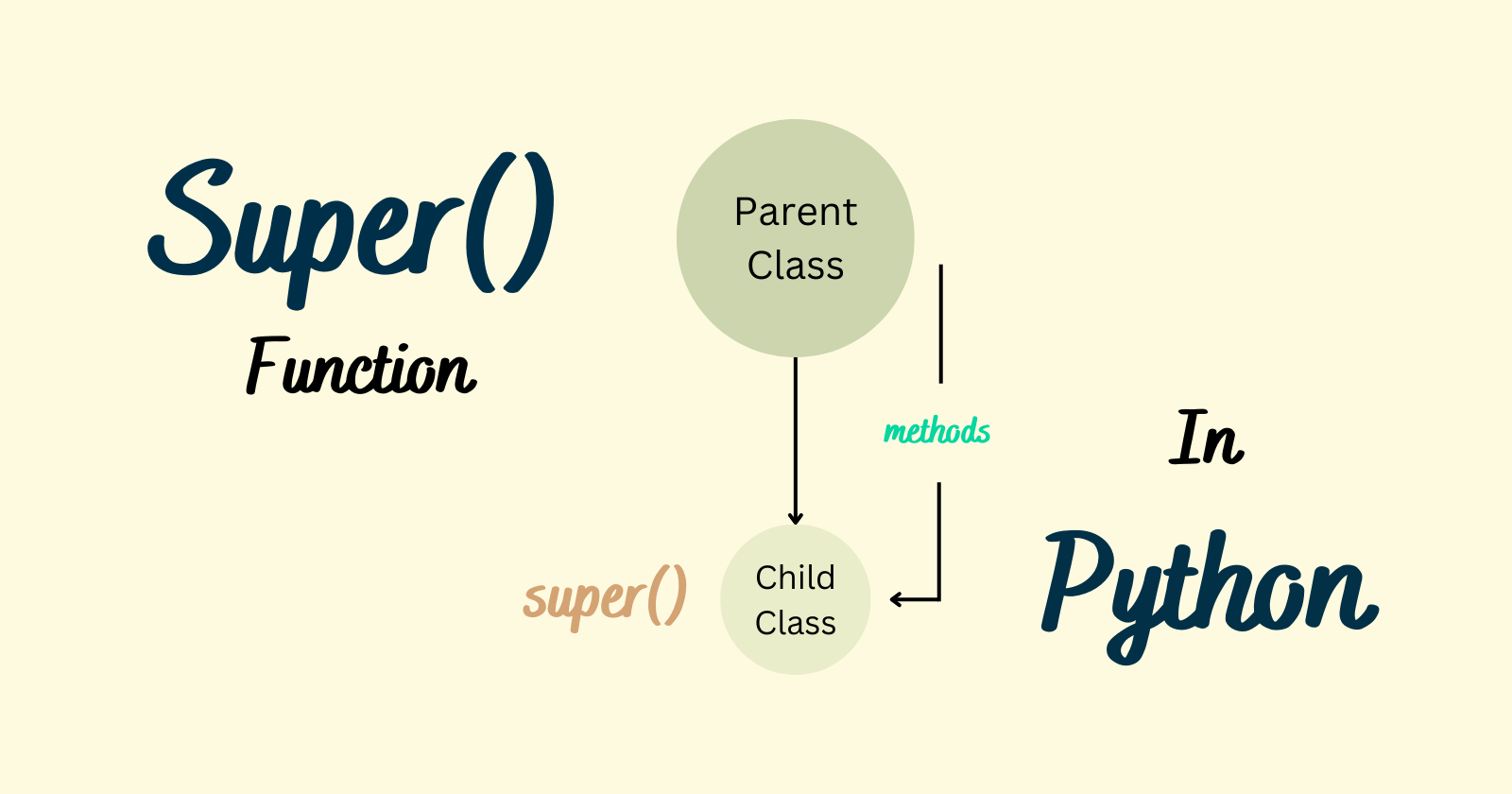## How To Use super() Function Within Python Classes

You may have heard the term inheritance in object-oriented programming, and if you haven’t, don’t worry because we’ve got your back.

Inheritance is one of the four pillars of object-oriented programming, and it can be defined as a mechanism that allows a specific class to inherit the attributes and methods of the parent class without having to implement them again.

Python has an excellent function called `super()` that allows the parent class’s attributes and methods to be fully accessible within a subclass.

What does the `super()` function do and how does it help with class inheritance in Python?

# Super in Python

To put it simply, the `super()` function extends the functionality of the superclass within the subclass or derived class. Assume we created a class and wanted to extend the functionality of a previously created class within that specific class; in that case, we’ll use the `super()` function.

Let’s look at an example to get a better understanding of the `super()` function in Python.

We made two classes, `BestFriend` and `Friend`, with the subclass or derived class `Friend` inheriting from the parent class `BestFriend`. However, in order to fully access the parent class within a derived class, we instantiated the parent class using the `super()` function.

## Syntax

The syntax of the `super()` function can be written as the following

Here:

`X` – is an optional parameter that represents the name of the subclass.

`self` – is the instance object of the derived class `X`.

`method` – is a normal function.

`args` – are the arguments of the function.

## What does the super() function do?

We saw earlier how to use the `super()` function within a subclass to extend the functionality of the parent class. And it’s no surprise that the `super()` function is mostly used to extend the functionality of the parent class within the subclass.

### super() function in single inheritance

Single inheritance is one in which the subclass or derived class inherits from a single parent class or superclass.

In the following example, there are two classes: `Triangle` and `RightTriangle`. Both classes have functions `area` that return the area of a triangle and a right-angle triangle.

Then we created instances of both classes, `triangle` and `rtriangle`, and passed them the necessary arguments. Then, using the respective instance variable, we called the function `area` of both classes.

We got the following output which was expected from the program.

However, by using inheritance and the `super()` function, we can reduce the amount of time we spend writing the same logic over and over. Let’s see how we can do it with the aforementioned code.

The class `RightTriangle` derives from the parent class `Triangle`, and we extended the functionality of the parent class within the subclass `RightTriangle` by using the `super()` function.

If we call the function `area` using the derived class `RightTriangle`, the code will run without error and we will get the correct output.

Because the derived class `RightTriangle` has access to the methods in the parent class `Triangle`, the code completed the task successfully.

### What else super() function can do?

We saw the basic usage of the `super()` function in the preceding segment, and we’ll see many more examples in this segment to help us understand more concepts of the `super()` function.

As we saw earlier, the `super()` function can take two parameters, but it can also be called without any parameters, as shown in the above code.

You can see that the derived class `RightTriangle` is the first parameter we passed, and the `RightTriangle` object `self` is the second. The code will continue to function flawlessly as before and won’t be at all impacted by this.

Calling `super(RightTriangle, self)` is equivalent to calling `super()` without parameters. In most cases, however, it is recommended that you use the parameter-free `super()` function.

Another subclass that inherits from the first subclass and extends the functionality of the parent class inside the second subclass can also use `super()`. Let’s use an example to better understand it.

The function `area`, which was originally inherited from the class `Rectangle` through the subclass `Square`, is extended by the subclass `Circle` in the example below to return the area of the circle.

If we run the aforementioned code, we will obtain the circle’s area calculated for a radius of 3.

### super() function in multiple inheritance

After seeing how the `super()` function is used in single inheritance, we will now use examples to show how it is used in multiple inheritance.

Multiple inheritance is a type of inheritance where a subclass inherits from various parent classes.

The class `Cylinder` in the example below inherits from the classes `Circle` and `Rectangle`. Like in the single inheritance example, we used the `area` function via the `super()` function.

The aim of the code is to print the area of the cylinder, and the function `cy_area` holds the logic to process it.

The code returns the above output, which is wrong, and this happens because the function `area` we called using the `super()` function is actually coming from the base class `Rectangle`.

If we look at the MRO (Method Resolution Order), that tells Python where to look for the inherited method.

Here, we can clearly see that Python will look for the inherited methods from the class `Rectangle,` and if the specific method is found in it, it will be used; otherwise, it will move ahead.

However, we want the variable `area` to access the function `area` via the class `Rectangle` and variable `area1` to access the function `area` via the class `Circle`.

You can change the order in which the base classes are inherited, though, if you ever need to use contradictory methods from classes within a subclass.

The solution to this problem is that we can modify the names of the function.

We changed the function’s name inside the class `Rectangle` and set it to `r_area`. We did the same inside the class `Circle` and set the function’s name to `c_area`. This gives the issue a short-term solution.

Note: When multiple inheritance is used, the order of the parent classes determines whether to use this class’ methods first or that class’ methods.

# Conclusion

We used the `super()` function in this tutorial to give subclasses full access to the parent classes’ methods and attributes. We first learned about the `super()` function through an example, and then we looked at the syntax.

Then, using code examples, we delve deep into the `super()` function to understand more concepts. Then we saw the use of `super()` in single and multiple inheritance, as well as a glimpse of the RMO, which is used in Python to resolve method calls.

🏆Other articles you might be interested in if you liked this one

That’s all for now

Keep Coding✌✌